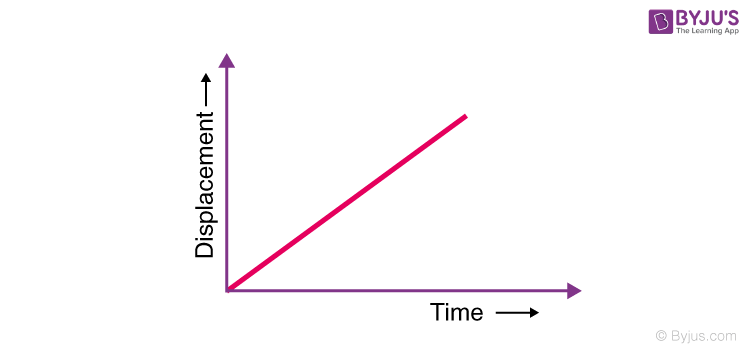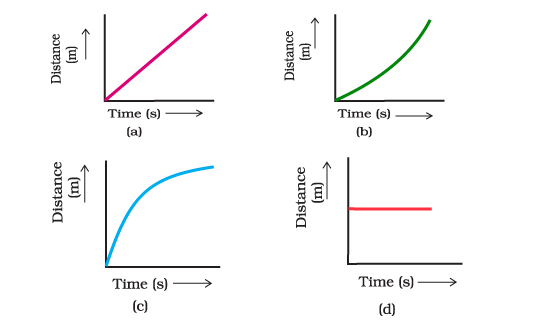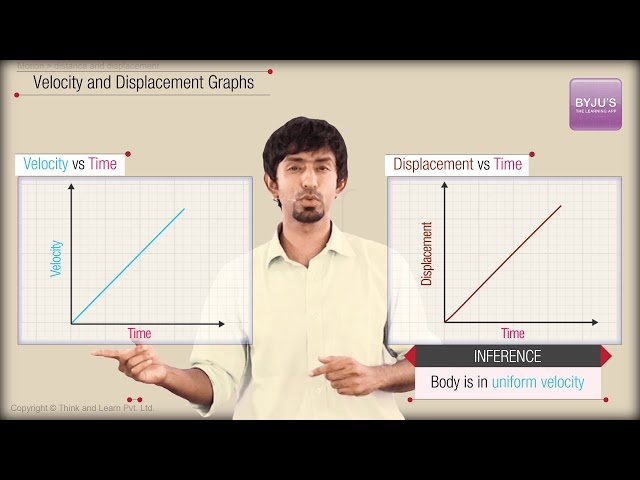# Distance Time Graph Questions

The graph is a two-dimensional drawing representing the relationship between independent and dependent variables. The study of graphs is known as graph theory. Each parameter is represented on the X-axis or Y-axis. The X-axis represents the independent variables, and the Y-axis represents the dependent variables.

A distance-time graph represents how far a body/object has travelled in a frame of a given time. It is the graph that represents distance and time. The time is plotted on the X-axis in the distance-time graph, and the distance is plotted on the Y-axis. The curved lines on a distance-time graph show that the speed is changing. When the distance and time for the motion of a body are recorded and plotted on a rectangular graph, the distance-time graph is obtained.

When the distance-time graph is a straight line, then the motion of the object is said to be uniform. The speed can be calculated using the slope of the distance-time graph. When the slope of the distance-time graph is the same irrespective of the interval which is chosen, this indicates that the speed of an object under uniform motion remains constant.

This graph is mainly used to calculate the distance from its initial point to the distance at which the body is at present. Time required to complete a definite distance can also be found. It can also be used to find the uniform motion speed. Variation in the movement of the body/object can also be determined by the body’s movement.

Important Distance Time Graph Questions with Answer

1. Choose YES or NO: Can the distance-time graph be used to represent the non-uniform motion of the body?

1. YES
2. NO

Explanation: The distance-time graph can indicate both the uniform and non-uniform motion of the body.

2. Which among the following graphs is not possible?

1. Displacement time graph
2. Time-time graph
3. Distance time graph
4. Velocity time graph

Explanation: We cannot plot the time-time graph since both parameters are the same.

3. In a distance-time graph, which variable is plotted on the Y-axis?

1. Velocity
2. Speed
3. Time
4. Distance

Explanation: In a distance-time graph, distance is plotted on the y-axis and time on the x-axis.

4. The below graph represents a distance-time graph with uniform or non-uniform velocity?Uniform velocity Non-uniform velocity

Explanation: When a body covers an equal distance in an equal interval of time, it is said to be in uniform velocity.

5. Which of the following options represents the distance-time graph of a stationary object?Explanation: Since a stationary object does not move, the graph is shown in option d) is the correct answer.

6. The slope of a distance-time graph represents _____

1. Velocity
2. Speed
3. Acceleration
4. Displacement

Explanation: Speed is indicated by the distance-time graph.

7. What are the three basic types of graphs?

Three basic types of graphs are:

• Line graphs
• Pie graphs
• Bar graphs

8. Motion of the blades of a ceiling fan represents which types of motion.

The motion of the blades of a ceiling fan represents uniform motion.

9. Distance-time graph shows a curved line in which type of motion?

Distance-time graph shows a curved line in non-uniform motion.

10. State true or false: A straight line on the distance-time graph indicates that the object is moving at a constant speed.

1. TRUE
2. FALSE